Next: Stereo Camera Calibration Up: Fitting Parameterized Models Previous: Grey model fitting

### Active Shape Model Location

One approach to locating examples of objects whose shape can vary (eg faces or internal organs) is to use Active Shape Models . This method relies on building a statistical model of shape variation from examples in a training set. Each example object is represented using a fixed number of landmark points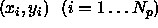, each of which marks a particular point on the object. The training examples are aligned into a common co-ordinate frame and each is then represented by a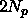element vector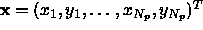. If we make the assumption that such vectors have a gaussian distribution for the training set we can build a linear model as follows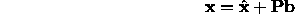where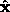is the mean of the training set, b is a vector of t shape parameters and P is ax t matrix formed from the t principle eigenvectors of the covariance matrix of the training set. The t shape parameters,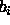, are then mutually independent and the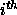is gaussian with zero mean and variance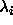(thelargest eigenvalue of the covariance matrix). See  for details.

For instance Figures 6 and 7 show the effects of varying two of the parameters of a face model which uses 169 points to represent the shape of various facial features.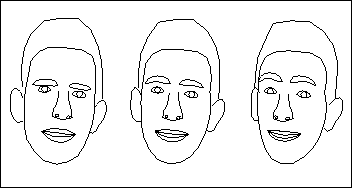Figure 6: Effect of varying shape parameter 1 of 169 point face model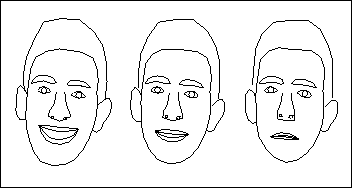Figure 7: Effect of varying shape parameter 2 of 169 point face model

To find the best fit of an instance of such a model to a new image, we must find the shape parameters, b, position,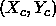, orientation,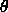, and scale, s, which best match the model to the image. Once again, defining a suitable objective function is difficult. The method used in the Active Shape Model is as follows.

We first must determine how well each individual model point matches the image, when placed at a particular location. For each model point we build a statistical model of the intensity variation in a region about each point, using the training set (section 3.2.1). This allows us to find a point in the image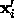which best matches themodel point, by searching a region around the current position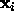. A good model position would have each point near to its corresponding best match, leading to an objective function of the form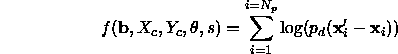where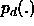is the distribution of location error. Because the model (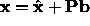) is a truncated approximation, it will not fit exactly to all plausible object shapes.

If we assume a gaussian distribution of point position errors, then a straightforward least squares method can be used to find the optimal parameters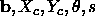for a given set of best match points.

Remember, however, that the best match points are obtained by searching around the current position for each model point. If the model points are not near the correct position then some of the matches will not be optimal, simply because the region searched did not include the true position for the point. The hope is that enough points were matched well, so that the new set of parameters moves the model points closer to the true solution. We thus use an iterative approach to solving the problem:

REPEAT

• Given parameterscalculate positions of model points,.
• Search in image around eachfor the best match of a corresponding region model,• Calculate the parameters which minimize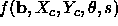UNTIL convergence.

When the starting point is `close enough' to the true image object, this algorithm will usually converge successfully. Its performance can be improved by using a multi-resolution framework in which the earlier iterations are performed using low resolution versions of the local region models searching on a low resolution version of the image . For instance, Figure 8 shows the face model iterating to fit a face image.

Notice that we could attempt to locate the optima by applying a local minimizer (eg Simplex) to. This would be very expensive since most of the work involved is in locating the best nearby matches for each model point, which would have to be done for every new function evaluation. The algorithm above attempts to make better use of the match information, updating the parameters effectively after every function evaluation. This approach of breaking a model matching problem into a series of independent local searches, followed by a parameter update (or regularization) stage is often used for Active Contour Models (`Snakes') and is discussed in detail by Cohen .

Notice that in the definition of the objective function only the position of the local model matches is used, not their quality. In theory, since we have a statistical model of the expected intensity variation for each match, we can assign a likelihood,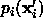, that the match is correct. We could then generate an objective function of the form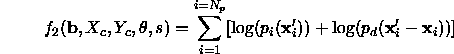However, this cannot be minimized using the two stage algorithm given above, and we must resort to the more expensive general methods.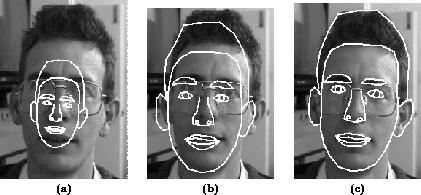Figure 8: a) Initial Position b) After 10 iterations c) At convergence of ASM searchNext: Stereo Camera Calibration Up: Fitting Parameterized Models Previous: Grey model fitting

Bob Fisher
Fri Mar 28 14:12:50 GMT 1997